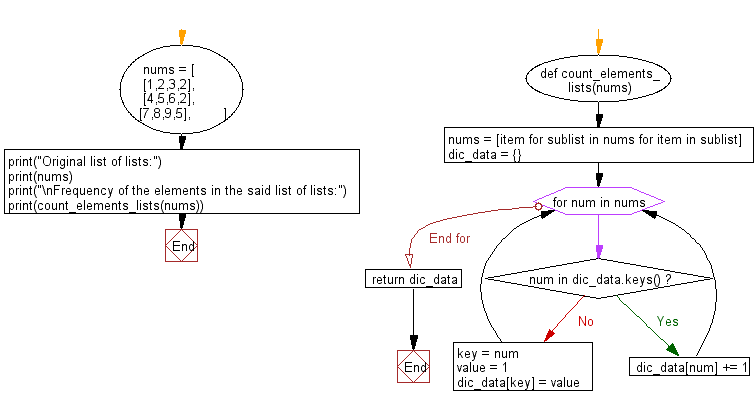﻿ Python: Frequency of the elements in a given list of lists - w3resource# Python: Frequency of the elements in a given list of lists

## Python List: Exercise - 143 with Solution

Write a Python program to get the frequency of the elements in a given list of lists.

Sample Solution:

Python Code:

``````def count_elements_lists(nums):
nums = [item for sublist in nums for item in sublist]
dic_data = {}
for num in nums:
if num in dic_data.keys():
dic_data[num] += 1
else:
key = num
value = 1
dic_data[key] = value
return dic_data

nums = [
[1,2,3,2],
[4,5,6,2],
[7,8,9,5],
]
print("Original list of lists:")
print(nums)
print("\nFrequency of the elements in the said list of lists:")
print(count_elements_lists(nums))
```
```

Sample Output:

```Original list of lists:
[[1, 2, 3, 2], [4, 5, 6, 2], [7, 8, 9, 5]]

Frequency of the elements in the said list of lists:
{1: 1, 2: 3, 3: 1, 4: 1, 5: 2, 6: 1, 7: 1, 8: 1, 9: 1}
```

Flowchart:## Visualize Python code execution:

The following tool visualize what the computer is doing step-by-step as it executes the said program:

Python Code Editor:

Have another way to solve this solution? Contribute your code (and comments) through Disqus.

What is the difficulty level of this exercise?

Test your Python skills with w3resource's quiz

﻿

## Python: Tips of the Day

```print(2_000_000)
```2000000